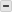# SUM Function

Sample Usage
SUM(Cost1:Cost5)
Syntax
SUM(
• number1
• [
• number2
• ...
]
)
• number1
The number or range of numbers to add
• number2
• ...
—[optional]
Examples

This example references the following sheet information:

Clothing Item Transaction Total Units Sold Price Per Unit
1Totals 4,285.00 337 250.00
2       T-Shirt 1,170.00 78 15.00
3       Pants 1,491.00 42 35.50
4       Jacket 812.00 217 200.00

Given the table above, here are some examples of using SUM in a sheet:

Formula Description Result
=SUM(12, 45, 72) Sums a series of numbers: 12, 45, and 72. 129
=SUM([Transaction Total]:[Transaction Total]) Sums the Transaction Total column  8,570.00
=SUM(CHILDREN()) Placed in the parent row to sum the child rows of the Transaction Total column. Rows 24 apply. (Row 1 is the parent row.) 4,285.00
=IF(SUM([Transaction Total]4)= ([Units Sold]4 * [Price Per Unit]4), "Total Correct" , "Total Incorrect") Returns the string Total correct if the value of row 4 in the Transaction Total column is equal to the product (amount when two numbers are multiplied by each other) of the value in row 4 of Units Sold and Price Per Unit columns. Otherwise, the function returns Total Incorrect. Total Incorrect

#### Still need help?

Use the Formula Handbook template to find more support resources, and view 100+ formulas, including a glossary of every function that you can practice working with in real time, and examples of commonly used and advanced formulas.

Find examples of how other Smartsheet customers use this function or ask about your specific use case in the Smartsheet online Community.